Unlimited Plugins, WordPress themes, videos & courses! Unlimited asset downloads! From \$16.50/m# Quick Tip: Get a Random Number Within a Specified Range Using AS3

This post is part of a series called You Do The Math.
Quick Tip: Trigonometry for Flash Game Developers

We often need to use a randomly generated number for certain situations; using Math.random() * n will usually do the trick, but it will only calculate a number from 0 to n. What if we need a number that doesn't give 0 as the minimum value? How can you generate a random number between 100 and 1000? I'll show you how to do it in this Quick Tip.

## Final Result

This example demonstrates the function we'll be creating:

Input two numbers separated by a ',' and press the random button.

## Step 1: Brief Overview

Using a function created in ActionScript 3, we will calculate a number between two values. These values will be passed as parameters and used with the Math class to generate a random number.

## Step 2: Create a New File

Open Flash and create a new Flash File (ActionScript 3.0).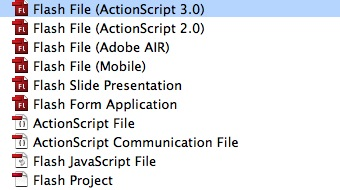## Step 3: Open the Actions Panel

Press Option + F9 or go to Window > Actions to open the Actions Panel.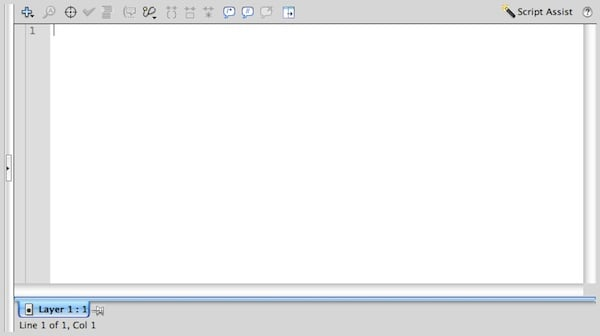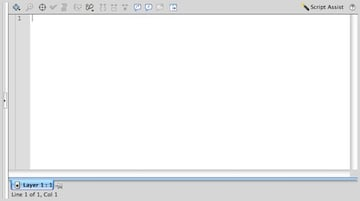## Step 4: Function Declaration

Declare a Function and name it randomRange; this function will return the random number, so set the return type to Number.

## Step 5: Set Parameters

Two parameters will be used to calculate the number.

• minNum: The minimum value to return
• maxNum: The maximum value to return

## Step 6: Write the Main Function

This is the function with the actual random number generator line. The power of Math is used to generate the number.

## Step 7: How it Works

We have our random number generator function, but what does this function do?

Take a look at the following image to get a better idea: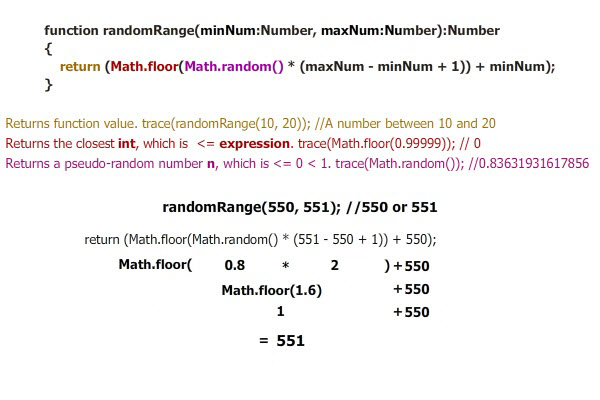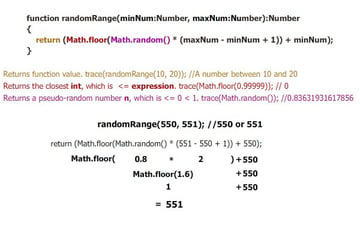In the image's example, if Math.random() was less than 0.5, the result would be 550.

## Step 8: Test with a Trace

A simple way to test the function is to use a trace() function. See the code below:

## Step 9: Example

This is a working example, it uses a button to calculate the number and display it in a TextField.

Input two numbers separated by a ',' and press the random button.

## Conclusion

This is a basic example of how you can use this function; experiment and use it in your own projects!